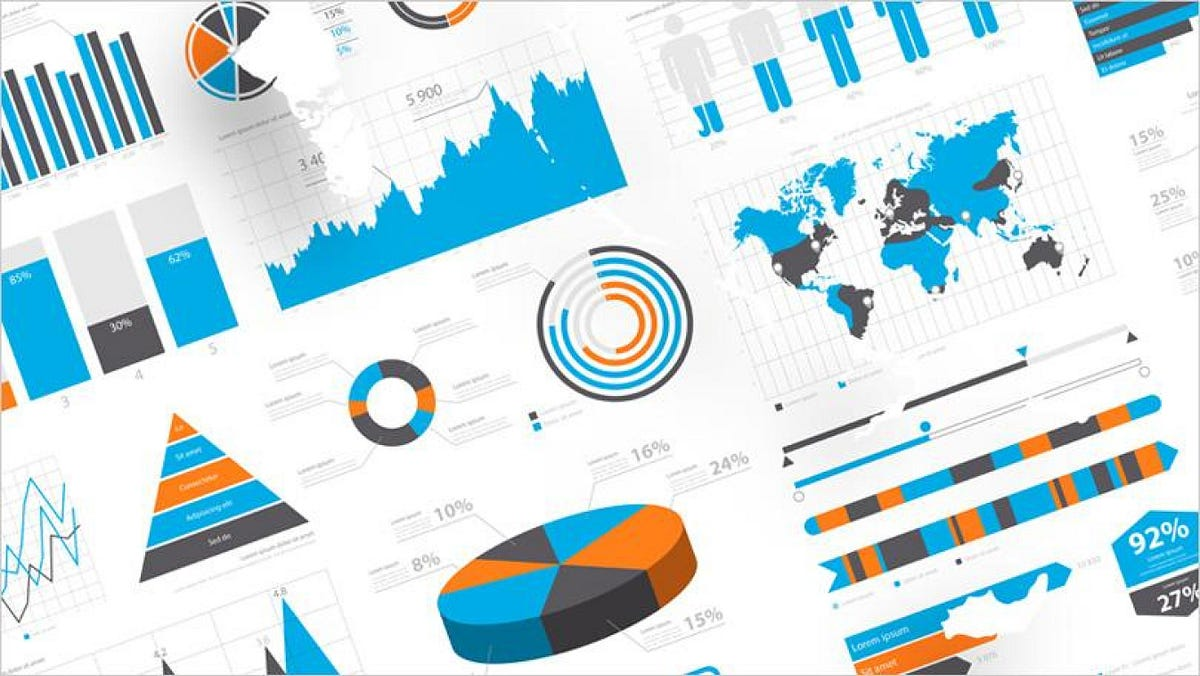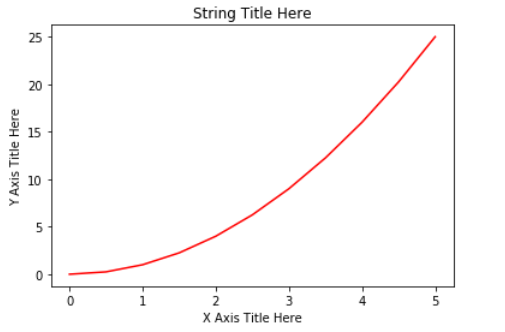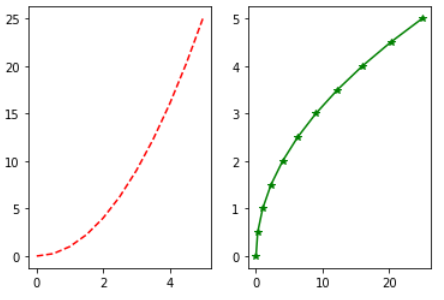# Data Visualization With Python: MatplotlibData visualization is the graphical representation of data in a graph, chart or other visual formats. It shows relationships of the data with images.

Data visualization is the graphical representation of data in a graph, chart or other visual formats. It shows relationships of the data with images.

Python offers multiple graphics libraries, with which you can create interactive, live or highly customizable plots with the given data.

To get a little overview here are a few popular plotting libraries:

In this article, we will learn about creating a different type of plots using the Matplotlib library.

Matplotlib is the most popular plotting library for python, which was designed to have a similar feel to MATLAB’s graphical plotting. It gives you control over every aspect of a plot.

Matplotlib allows you to create reproducible figures using a few lines of code. Let’s learn how to use it! I also encourage you to explore: http://matplotlib.org/.

## Installing Matplotlib

Install it with pip or conda at your command line or the terminal with:-

``````pip install matplotlib
OR
conda install matplotlib``````

To quickly get started with Matplotlib without installing anything on your local machine, check out Google Colab. It provides Jupyter Notebooks hosted on the cloud for free which are associated with your Google Drive account and it comes with all the important packages pre-installed.

## Necessary Imports

`pyplot` is a module of Matplotlib that makes this library work like MATLAB. Import the `matplotlib.pyplot` module under the name `plt` (the tidy way):

``````import matplotlib.pyplot as plt
import numpy as np # for working with arrays ``````

## Making a Simple Plot

We pass two NumPy arrays(x and y) and ‘r’ as arguments to Pyplot’s `plot()` function. Here ‘r’ is for red colour, x elements will appear on x-axis and y elements will appear on the y-axis.

``````import matplotlib.pyplot as plt
import numpy as np

x = np.array([0, 0.5, 1, 1.5, 2, 2.5, 3, 3.5, 4, 4.5, 5])
y = x ** 2 # y is now a list with elements of x to the power 2

plt.plot(x, y, 'r')
plt.xlabel('X Axis Title Here')
plt.ylabel('Y Axis Title Here')
plt.title('String Title Here')
plt.show()
# The plot below is the output of this program.``````Creating Multiple Plots on The Same Canvas

`subplot()`: a method of pyplot, divides the canvas into `nrows` x `ncols` parts and using `plot_number` argument you can choose the plot.

Syntax: `subplot(nrows, ncols, plot_number)`

In the below example, using `plt.plot(x, y, 'r--’)`we plot a red coloured graph with line style ‘- -’ between x and y at plot_number=1.

``````import matplotlib.pyplot as plt
import numpy as np

x = np.array([0, 0.5, 1, 1.5, 2, 2.5, 3, 3.5, 4, 4.5, 5])
y = x ** 2
plt.subplot(1,2,1) # subplot(nrows, ncols, plot_number)
plt.plot(x, y, 'r--') # r-- meaning colour red with -- pattern
plt.subplot(1,2,2)
plt.plot(y, x, 'g*-') # g*- meaning colour green with *- pattern
# The plot below is the output of this program.``````For making it more simple `subplots()` method can be used instead of `subplot()`. You will see its example in “Creating Multiple plots on The Same Canvas” under “Matplotlib Object-Oriented Method”.

## Data Visualization with Matplotlib in Python (Part 1)

So here is my first blog regarding the data visualization with matplotlib in python. In this article we will cover the basic of the visualization with matplotlib.

## Data Visualization With Python | Data Visualization | Python For Data Science

🔥To access the slide deck used in this session for Free, click here: https://bit.ly/GetPDF_DataV_P 🔥 Great Learning brings you this live session on 'Data Vis...

## Applied Data Science with Python Certification Training Course -IgmGuru

Master Applied Data Science with Python and get noticed by the top Hiring Companies with IgmGuru's Data Science with Python Certification Program. Enroll Now

## An introduction to exploratory data analysis in python

Many a time, I have seen beginners in data science skip exploratory data analysis (EDA) and jump straight into building a hypothesis function or model. In my opinion, this should not be the case.

## Exploratory Data Analysis is a significant part of Data Science

Data science is omnipresent to advanced statistical and machine learning methods. For whatever length of time that there is data to analyse, the need to investigate is obvious.